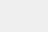# 外汇中的点差是怎么算的

USDCHF的点值=100000*0.0001*（CHF/USD）=10/USD/CHF=10/0.9245=10.82美金

USDJPY的点值=100000*0.01*（JPY/USD）=1000/USD/JPY=1000/81.6775=12.24美金

EURGPB的点值=100000*0.0001*（GPB/USD）=10*1.61=16.1美金

GPBCHF的点值=100000*0.0001*（CHF/USD）=10/USD/CHF=10/0.9245=10.82